### THE METRIC TENSOR: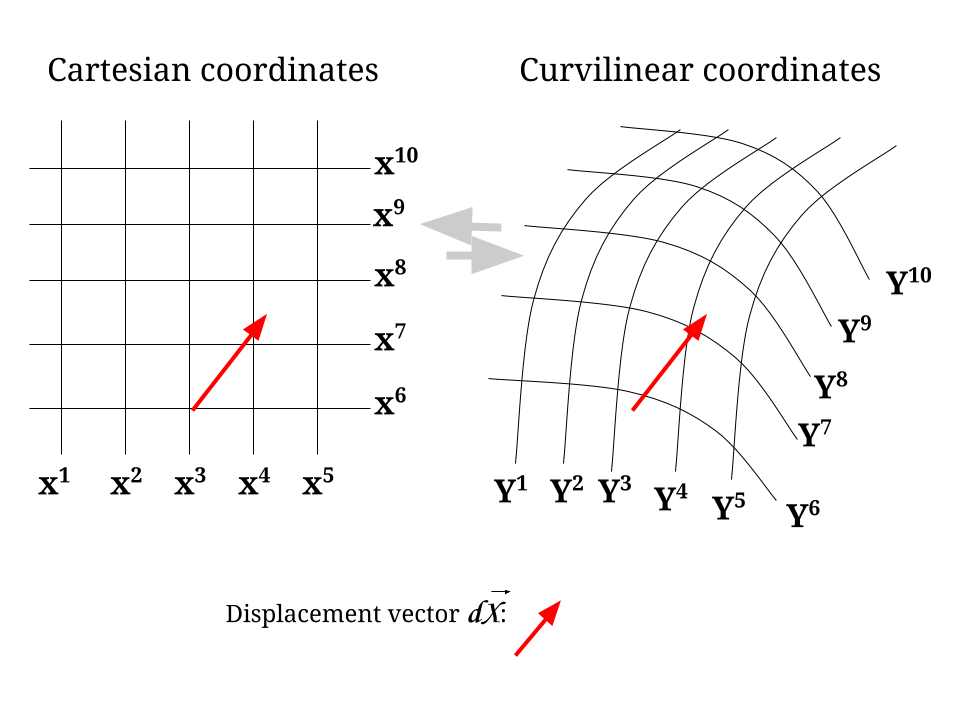The length or magnitude of the vector $$dS$$ in Cartesian coordinates is given by:

$dS^2=\left(dX^1\right)^2 + \left(dX^2\right)^2 + \left(dX^3\right)^2 + \cdots = \large \delta_{mn} \;\color{blue}{dX^m}\, dX^n\tag 1$

Notice that the dummy indexes are set up to sum over. We start with $$m=1$$, and we see that all terms are going to be zero unless when $$n=1$$, in which case it will be $$dX^1\, dX^1 = (dX^1)^2.$$

But how to write the same for the curvilinear system?

We have to refer back to the following expression of a change of coordinate system at the beginning of the notes on tensors:

$dy^n = \frac{\partial y^n}{\partial x^\color{blue}{m}} dx^{\color{blue}{m}} \tag{Ref.1}$

In this case we have that the part in blue in Eq. 1 can be expressed as:

$\color{blue}{dX^m}= \frac{\partial x^m}{\partial y^r} dy^r \tag2$

Hence, Eq. 1 becomes,

$dS^2 = \large \delta_{mn} \;dX^m\, dX^n= \large \underset{\text{METRIC TENSOR }\Large g^{(y)}_{\color{red}{rs}}}{\underbrace{\delta_{mn}\frac{\partial x^m}{\partial y^\color{red}{r}}\frac{\partial x^n}{\partial y^\color{red}{s}}}} \;dy^r\; dy^s$

$\huge \bbox[10px, border:2px solid aqua]{g^{(y)}_{\color{red}{rs}}=\delta_{mn}\frac{\partial x^m}{\partial y^\color{red}{r}}\frac{\partial x^n}{\partial y^\color{red}{s}}}\tag 3$

Here is another derivation: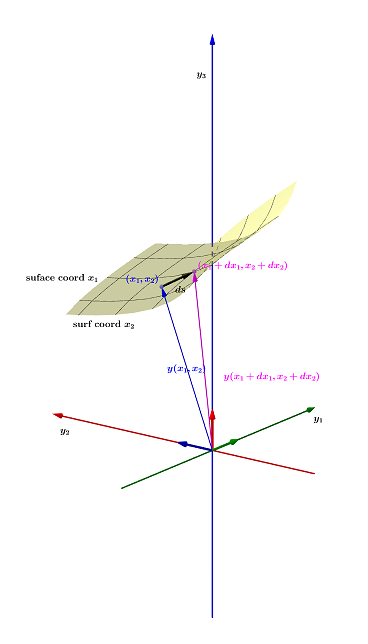We can define the position vector $$y$$ in terms of the surface coordinates $$x_1$$ and $$x_2.$$

Substracting the shifted vector along one of the coordinates from the original one we end up with a tangent vector:

$\frac{y(x_1 + dx_1, x_2)- y(x_1,x_2)}{dx_1}=\vec e_1$ The same applies to $$\vec e_2:$$

$\frac{y(x_1, x_2+dx_2)- y(x_1,x_2)}{dx_2}=\vec e_2$

So the $$\vec e_1$$ and $$\vec e_2$$ is a basis for the tangent space at the point $$y(x_1,x_2).$$

Expanding the Taylor series of the position vector for the translated point:

\begin{align} y(x_1 + dx_1, x_2 + dx_2) &= y(x_1, x_2) + \frac{\partial y}{\partial x_1}dx_1+ \frac{\partial y}{\partial x_2}dx_2\\[2ex] &= y(x_1,x_2) + \vec e_1 dx_1 + \vec e_2 dx_2 \end{align}

The difference in position vectors gives a distance vector:

$d\vec s= y(x_1 + dx_1, x_2 + dx_2) - y(x_1,x_2)=\vec e_1 dx_1 + \vec e_2 dx_2$

and the square distance is (changing to covariant/contravariant notation) $d\vec s \cdot d\vec s = (\vec e_1 dx^1 + \vec e_2 dx^2)\cdot (\vec e_1 dx^1 + \vec e_2 dx^2)=\sum_{i=1}^2 \sum_{j=1}^2 g_{ij}dx^idx^j$

with $$g_{ij}=\vec e_i \cdot \vec e_j$$ being the metric tensor waiting for two vectors to produce a scalar. It can be represented as a symmetric matrix with dim equal to the number of intrinsic coordinates:

$ds^2 = \begin{bmatrix}dx_1& dx_2\end{bmatrix}\color{blue}{\begin{bmatrix}g_{11}&g_{12}\\g_{21}&g_{22}\end{bmatrix}}\begin{bmatrix}dx_1\\dx_2\end{bmatrix}$ which is exactly the same operation as the (0,2) tensor presented here:

$g_{\mu\nu}\,dx^\mu\otimes dx^\nu=g_{\mu\nu}(x)\,dx^\mu\otimes dx^\nu= \sum_{i=1}^2 \sum_{j=1}^2 g_{ij}dx^idx^j=\begin{bmatrix}dx_1& dx_2\end{bmatrix}\color{blue}{\begin{bmatrix}g_{11}&g_{12}\\g_{21}&g_{22}\end{bmatrix}}\begin{bmatrix}dx_1\\dx_2\end{bmatrix}$ with $$g_{\mu\nu}(x)$$ indicating that it is a function of the space time (depends on the point on the manifold).

Of note, the metric tensor is the identity matrix in Cartesian coordinates:

$ds^2 = \begin{bmatrix}dx_1& dx_2\end{bmatrix}\color{blue}{\begin{bmatrix}1&0\\0&1\end{bmatrix}}\begin{bmatrix}dx_1\\dx_2\end{bmatrix}$

We write,

$dS^2 = q^{(y)}_{rs}\,dy^r\,dy^s$

If we were operating with both systems being curvilinear, the measure of the vector wouldn’t be as simple in the $$X$$ system as before. We would have:

$\large g^{(x)}_{mn}\,dx^m\,dx^n=g^{(y)}_{rs}\,dy^r\,dy^s$

applying Eq. 2,

$\large g^{(x)}_{mn}\frac{\partial x^m}{\partial y^r}\frac{\partial x^n}{\partial y^s}dy^r\,dy^s= g^{(y)}_{rs}\,dy^r\,dy^s$

$\large g^{(x)}_{mn}\frac{\partial x^m}{\partial y^r}\frac{\partial x^n}{\partial y^s} = g^(y)_{(rs)}$

which is a covariant transformation. So the matrix tensor does transform like a covariant tensor.

From Tensors:

$\bbox[10px, border:2px solid aqua]{ \varepsilon_i =\frac{\partial \vec r}{\partial u^i}}$

we have that a displacement vector $$\vec r$$ in a curvilinear system of coordinates: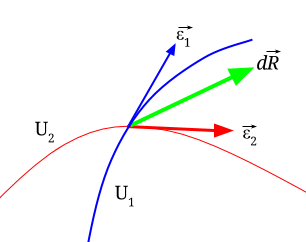$\large d\vec r = \vec \varepsilon_1 d u^1 + \vec \varepsilon_2 d u^2 + \cdots = \vec \varepsilon_i du^i$

$\large dS^2 = d\vec r \cdot d\vec r$

$\large dS^2 = \vec e_i \,du^i \cdot \vec e_j\,du^j=\vec e_i \, \vec e_j \, du^i \, du^j$

$\large dS^2 = g_{ij}\, du^i\, du^2$

which implies that the dot product of two tangent vectors is the metric tensor:

$\huge\bbox[10px, border:2px solid blue]{\vec \varepsilon_i\vec \varepsilon_j = g_{ij}}\tag 4$

Of note, for expressions in terms of COVARIANT COMPONENTS we have the CONTRAVARIANT FORM of the metric tensor:

$\huge\bbox[10px, border:2px solid blue]{\vec \varepsilon^i\vec \varepsilon^j = g^{ij}}\tag {CONTRAVARIANT FORM}$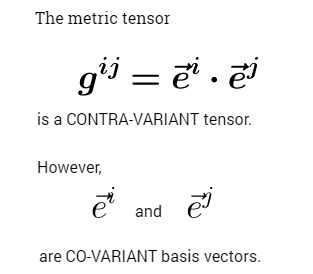NOTE: DO NOT CONFUSE WITH $$e_i\,e^j =\delta_i^j$$ or $$e^i\,e_j= \delta_j^i$$ (Eq. 11 under tensors), which simply expresses that tangent vectors are orthogonal to orthogonal vectors in the generalized curvilinear system.

KEY CONCEPT: The dot product of two unit tangent vectors (contravariant basis - subindices in basis; supraindeces in vectors) is the metric tensor (same goes for the dot product between orthogonal vectors (covariant basis)), whereas the dot product between unit tangent and orthogonal vectors is the Kronecker delta.

Looking at cylindrical coordinates: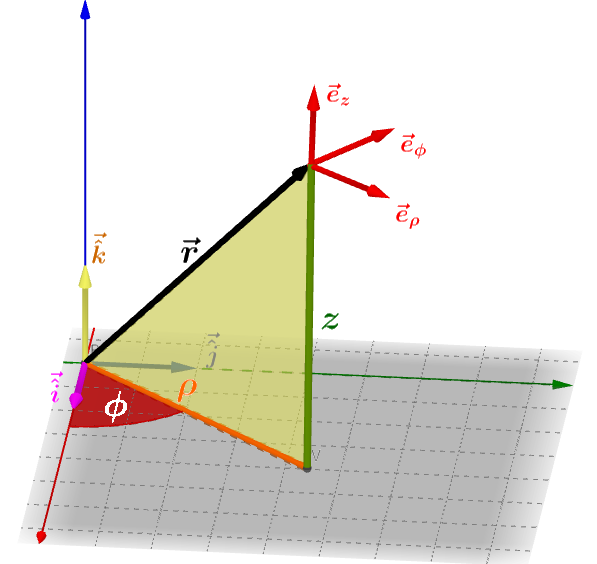$\vec r = \rho\, \cos(\phi) \vec {\hat i} + \rho\, \sin(\phi)\vec {\hat j} + z \vec {\hat k}$

$\vec e_\rho = \vec e_1 = \frac{\partial \vec r}{\partial \rho}= \cos(\phi) \vec {\hat i} + \sin(\phi) \, \vec{\hat j}$

$\vec e_\phi = \vec e_2 = \frac{\partial \vec r}{\partial \phi}= -\rho \sin(\phi) \vec {\hat i} + \rho\,\cos(\phi)\,\vec{\hat j}$

$\vec e_z = \vec e_3= \frac{\partial \vec r}{\partial z}= \vec{\hat k}$

From Eq. 4, $$g_{ij}= \vec e_i \vec e_j$$, we have

$\begin{matrix}\vec e_1\vec e_1&\vec e_1\vec e_2&\vec e_1\vec e_3\\ \vec e_2\vec e_1&\vec e_2\vec e_2&\vec e_2\vec e_3\\ \vec e_3\vec e_1&\vec e_3\vec e_2&\vec e_3\vec e_3 \end{matrix}$

So let’s take the element $$g_{11}=(1,1)=(\vec e_1\vec e_1):$$

$g_{11}= \left( \cos(\phi) \vec {\hat i} + \sin(\phi ) \, \vec{\hat j}\right)\left( \cos(\phi) \vec {\hat i} + \sin(\phi) \, \vec{\hat j}\right)= \cos^2(\phi)+\sin^2(\phi)=1$

Notice that $$\vec {\hat i}\cdot \vec {\hat j}=0.$$

Moving to $$g_{12}=0$$, which means that $$g_{21}=0.$$

What about $$g_{22}=\left(-\rho \sin(\phi) \vec {\hat i} + \rho\,\cos(\phi)\,\vec{\hat j}\right)\left(-\rho \sin(\phi) \vec {\hat i} + \rho\,\cos(\phi)\,\vec{\hat j}\right)=\rho^2\sin^2(\phi)+\rho^2\cos^2(\phi)=\rho^2$$

And $$g_{23}=0$$; $$g_{13}=0$$; and $$g_{33}=1$$

Hence the metric tensor for cylindrical coordinates is:

$g_{ij}=\begin{bmatrix}1&0&0\\0&\rho^2&0\\0&0&1 \end{bmatrix}$

which makes sense, because we have orthogonal tangent vectors in this particular case, explaining that the only non-zero entries are in the diagonal. But in a more general curvilinear system, the tangent vectors will not be orthogonal to each other.

RAISING AND LOWERING INDEXES:

Let’s consider a vector $$\vec A$$ in contravariant $$(\vec A = a^1\,\vec e_1 + a^2\,\vec e_2 + a^3\, \vec e_3+\cdots = a^i\,\vec e_i)$$, and covariant coordinates $$(\vec A = a_1\,\vec e^1 + a_2\,\vec e^2 + a_3\, \vec e^3+\cdots = a_i\,\vec e^i)$$. Similarly, we have a vector $$\vec B$$ also expressed in terms of contra and covariant components.

We know that $$e_i\,e^j =\delta_i^j$$ or $$e^i\,e_j= \delta_j^i$$ (Eq. 11 under tensors).

Taking the dot product of $$\vec A$$ and $$\vec B$$, we can get four different expressions:

1. Contravariant components of A and B:

$\vec A\vec B= a^ie_i\cdot b^je_j= a^ib^j e_i\cdot e_j = g_{ij}a^ib^j$ with $$g_{ij}$$ being the covariant form of the tensor matrix.

1. Covariant components of A and B:

$\vec A\vec B= a_ie^i\cdot b_je^j= a_ib_j e^i\cdot e^j = g^{ij}a^ib^j$ with $$g^{ij}$$ being the contravariant form of the tensor matrix.

1. Covariant components of A and contravariant components of B:

$\vec A\vec B= a_ie^i\cdot b^je_j= a_ib^j e^i\cdot e_j = \delta_j^i a_i b^j = a_ib^i$ with $$e^i\cdot e_j =\delta_j^i$$ (see comment or note under Eq. 4 above).

1. Contravariant components of A and covariant components of B:

$\vec A\vec B= a^ie_i\cdot b_je^j= a^ib_j e_i\cdot e^j = \delta_i^j a^i b_j = a^ib_i$

So, what happens if we equate the results in entry (1) with entry (4):

$\Large g_{ij}a^ib^j = a^ib_i$

and

$\require{cancel}\Large g_{ij}\cancel{a^i}b^j = \cancel{a^i}b_i$

$\Large \color{red}{g_{ij} b^j = b_i}$

so $$b^j$$ is a contravariant component of a vector, which gets transformed into the covariant component of the vector when operating with the covariant metric tensor. We can think of it like the dummy indeces $$j$$ anihilate each other.

If instead we equate the results in (2) and (3),

$\Large g^{ij}a_ib_j = a_ib^i$

$\Large g^{ij}\cancel{a_i} b_j = \cancel{a_i}b^i$

$\Large \color{blue}{g^{ij} b_j = b^i}$

so $$b_j$$ is a covariant component of a vector, which gets transformed into the contravariant component of the vector when operating with the contravariant metric tensor.

Now, since $$g^{ij}b_j=b^i$$, and $$\delta_k^i b^k = b^i$$,

$\Large g^{ij} \color{red}{b_j} = b^i = \delta_k^i b^k$

and replacing $$b_j$$ for the expression in red above:

$\Large g^{ij} \color{red}{b_j} = g^{ij} \color{red}{g_{jk}b^k} = b^i = \delta_k^i b^k$

Therefore,

$\Large \delta_k^i b^k = g^{ij} \color{red}{g_{jk}b^k}$

which implies

$\Large \bbox[10px, border:2px solid blue]{g^{ij}\Large g_{jk}= \delta_k^i}$

Remembering now that these are matrices, we see that,

$\Large\delta_k^i=\begin{bmatrix}1&0&0\\0&1&0\\0&0&1 \end{bmatrix} = I$

And remembering that

$g_{ij}=\begin{bmatrix}1&0&0\\0&\rho^2&0\\0&0&1 \end{bmatrix}$

we have to conclude that $$g^{ij}$$ is the inverse of $$g_{ij}$$, and as a corollary we can conclude that $$g_{ij}$$ and $$g^{ij}$$ are invertible. $$g^{ij}$$ is the inverse of $$g_{ij}.$$

$g^{ij} = \begin{bmatrix}1&0&0\\0&1/\rho^2 &0\\0&0&1 \end{bmatrix}$

#### TRANSFORMATION OF METRIC TENSORS:

Starting with equation (4) above,

$\vec \varepsilon_i\vec \varepsilon_j = g_{ij}$

transformations of this metric tensor between two systems of curvilinear coordinates, where the expression of this metric tensor in the second system is $$g'_{ij}= \vec \varepsilon'_i \varepsilon'_j$$ can be derived from Equation (ref.2 and ref.3) in Tensors

$\bbox[10px,border:2px solid purple]{\large \vec \varepsilon'_i = \frac{\partial u^{j}}{\partial u^{'i}} \vec \varepsilon_j}\tag {ref.3}$

Let’s re-write this as $$\large \vec e'_i=\frac{\partial u^k}{\partial u'^i}\vec e_k$$, expressing the change between coordinates of a tangential vector component. For $$\varepsilon_j$$ in equation (4) we can write an equivalent expression $$\large \vec e'_j=\frac{\partial u^l}{\partial u'^j}\vec e_l.$$

Now substituting in equation (4), we can derive:

$\large g'_{ij} = \frac{\partial u^k}{\partial u'^i}\frac{\partial u^l}{\partial u'^j}\color{red}{\vec e_k\cdot\vec e_l}$

Now, $$g_{kl}=\color{red}{\vec e_k\cdot\vec e_l}$$, and hence:

$\large g'_{ij}= \frac{\partial u^k}{\partial u'^i}\frac{\partial u^l}{\partial u'^j} g_{kl}\tag 5$

This is a covariant transformation - metric tensors with subscrips transform as covariant tensors.

On the other hand, for

$\large g'^{ij} = \frac{\partial u'^i}{\partial u^k}\frac{\partial u'^j}{\partial u^l}g^{kl}$

we observe a contravariant transformation.

#### CHRISTOFFEL SYMBOLS:

The tangential vectors are the result of $$\large \vec\varepsilon_i = \frac{\partial \vec r}{\partial u^i}$$ so that in the case of spherical coordinates we have the basis vectors (tangential vectors) expressed as the partial derivatives of $$\large \vec r = r\, \sin\theta\cos\phi\, \vec {\hat e_1} + r\, \sin\theta\sin\phi\, \vec{\hat e_2} + r\, \cos\theta\, \vec {\hat e_3}$$:

$\vec \varepsilon_r = \frac{\frac{\partial \vec r}{\partial r}}{\lVert\frac{\partial \vec r}{\partial r}\rVert}= \sin\theta\cos\phi \vec {\hat e_1} + \sin\theta\sin\phi \vec {\hat e_2} + \cos\theta \vec {\hat e_3}$

$\vec \varepsilon_\theta = \frac{\frac{\partial \vec r}{\partial \theta}}{\lVert\frac{\partial \vec r}{\partial \theta}\rVert}= r \cos\theta\cos\phi \vec {\hat e_1} +r \cos\theta\sin\phi \vec {\hat e_2} - r\sin\theta \vec {\hat e_3}$

$\vec \varepsilon_\phi = \frac{\frac{\partial \vec r}{\partial \phi}}{\lVert\frac{\partial \vec r}{\partial \phi}\rVert}= -r \sin\theta\sin\phi \vec {\hat e_1} +r \sin\theta\cos\phi \vec {\hat e_2}$

so that in general, any vector $$\vec A$$ can be written as:

$\large \vec A= a^1\,\vec{\varepsilon_1}+ a^2\,\vec{\varepsilon_2} + a^3\,\vec{\varepsilon_3}$

or more succintly,

$\large \vec A = a^k\, \vec \varepsilon_k$

What if we took second derivatives of these tangential basis?

So we can write this vector resulting of these second derivatives of the basis vectors also in terms of the basis vectors (tangentials):

$\large\frac{\partial \vec \varepsilon_i}{\partial u^j} = \beta^k \vec {\varepsilon_k}=\Large \underset{\text{Christoffel symbol}}{\underbrace{\Gamma_{ij}^k}} \vec \varepsilon_k$

and we can think of the Christoffel symbol as the vector that we obtain by taking the partial derivative of one of the basis vectors (tangents) expressed on the same basis vectors in the curvilinear system.

Now let’s retieve the concept of reciprocal basis, which ended up with the expression $$\large \varepsilon_i \varepsilon^j =\delta_i^j$$ to see that

$\frac{\partial \vec \varepsilon_i}{\partial u^j} \cdot \vec \varepsilon^k = \Gamma_{ij}^k \vec \varepsilon_k\cdot\vec\varepsilon^k = \Gamma_{ij}^k\delta_k^k =\Gamma_{ij}^k$

Ahaaa… So

$\Gamma_{ij}^k= \frac{\partial \vec \varepsilon_j}{\partial u^j}\cdot\vec \varepsilon^k$

Notice that 3D space $$k=1,2,3$$ and the same goes for $$i$$ and $$j$$. So the Christoffel symbol can have $$27$$ different components.

$\large \color{red}{\large \frac{\partial \vec \varepsilon_i}{\partial u^j}}= \frac{\partial \vec r}{\partial u^j\partial u^i}= \frac{\partial \vec r}{\partial u^i \partial u^j}=\frac{\partial \vec \varepsilon_j}{\partial u^i}$

Now,

$\large \color{red}{\large \frac{\partial \vec \varepsilon_i}{\partial u^j}}=\Gamma_{ij}^k \vec \varepsilon_k= \Gamma_{ji}^k\vec\varepsilon_k$

Hence,

$\large \Gamma_{ij}^k = \Gamma_{ji}^k$

much like $$\large g_{ij}=g_{ji}= \vec \varepsilon_j\cdot\vec\varepsilon_i.$$

Let’s consider taking a partial derivative of the metric tensor:

$\large \frac{\partial g_{ij}}{\partial u^k}= \frac{\partial \vec \varepsilon_i}{\partial u^k}\cdot\vec\varepsilon_j + \vec \varepsilon_i \frac{\partial \vec \varepsilon_j}{\partial u^k}$

We can now use Christoffel symbols:

$\large \frac{\partial g_{ij}}{\partial u^k}=\Gamma_{ik}^l \vec\varepsilon_l\cdot\vec\varepsilon_j+ \vec \varepsilon_i \cdot \Gamma_{jk}^l\vec\varepsilon_l$

If we look into this last expression we have two metric vectors: $$\large g_{lj}= \vec\varepsilon_l\cdot\vec\varepsilon_j$$ and $$g_{il}= \vec\varepsilon_i\cdot\vec\varepsilon_l$$. Hence,

$\large \frac{\partial g_{ij}}{\partial u^k}= \color{blue}{\Gamma_{ik}^l \;g_{lj}}+\color{red}{\Gamma_{jk}^l \;g_{il}}$

Next step, we reshuffle the indexes:

$\large \frac{\partial g_{jk}}{\partial u^i}= \color{purple}{\Gamma_{ji}^l \;g_{lk}}+\color{blue}{\Gamma_{ki}^l \;g_{jl}}$

$\large \frac{\partial g_{ki}}{\partial u^j}= \color{red}{\Gamma_{kj}^l }\;g_{li}+\color{purple}{\Gamma_{ij}^l \;g_{kl}}$

OK… We add the last two equations and subtract the one on top, and noting the color-coded identities,

$\large 2\,\Gamma_{ij}^l\,g_{kl}=\frac{\partial g_{ki}}{\partial u^j}+\frac{\partial g_{jk}}{\partial u^i}-\frac{\partial g_{ij}}{\partial u^k}$

or

$\large \Gamma_{ij}^l\,g_{kl} = 1/2 \left[ \frac{\partial g_{ki}}{\partial u^j}+\frac{\partial g_{jk}}{\partial u^i}-\frac{\partial g_{ij}}{\partial u^k}\right]$

Remembering that $$\large g_{kl}\,g^{mk}=\delta_l^m$$,

$\large \Gamma_{ij}^l\,g_{kl}\,g^{mk} = 1/2\,g^{mk}\,\left[ \frac{\partial g_{ki}}{\partial u^j}+\frac{\partial g_{jk}}{\partial u^i}-\frac{\partial g_{ij}}{\partial u^k}\right]$

$\large \Gamma_{ij}^l\,\delta_l^m = 1/2\,g^{mk}\,\left[ \frac{\partial g_{ki}}{\partial u^j}+\frac{\partial g_{jk}}{\partial u^i}-\frac{\partial g_{ij}}{\partial u^k}\right]$

which implies

$\large \Gamma_{ij}^m = 1/2\,g^{mk}\,\left[ \frac{\partial g_{ki}}{\partial u^j}+\frac{\partial g_{jk}}{\partial u^i}-\frac{\partial g_{ij}}{\partial u^k}\right]$